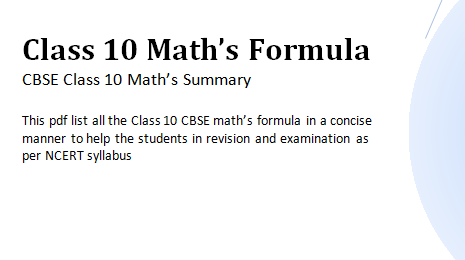## What is Similarity in math (geometry)?

Similarity – Geometry (basics and concept:- similarity math) Geometry is a branch of mathematics. While studying geometry we are concerned with questions of shape, size, the relative position of figures, and the properties of space. This article is about similarity (geometry) as we study in math. The figure below shows various figures having the same color are similar figures. We often come across objects that … Continue reading What is Similarity in math (geometry)? »

## Arithmetic progression questions for class 10

This page contains arithmetic progression questions for class 10. In this link get short arithmetic progression class 10 notes. Few other links are arithmetic progression class 10 important questions arithmetic progression assignment Practicing these questions on Arithmatic progression for class 10 maths can be helpful for your board exams. Arithmetic Progression questions and answers class 10 maths One Marks Questions Question 1. If k, 2k … Continue reading Arithmetic progression questions for class 10 »

Trigonometry is quite a interesting subject. Some of the basic in trigonometry which need to be remember is

tan(x) = sin(x)/cos(x)

cot(x) = cos(x)/sin(x)

Reciprocal Identities:

csc(x) = 1/sin(x)

## CBSE sample paper for class 10

This page consists of lot of cbse sample papers for class 10 for both maths and Science. So all the best for your exams. If you like the content do not forget to like share or comment at the section given below. you will find extra resource links (science study material) at the end of the page.   CBSE Science sample paper for class 10(2017-2018) … Continue reading CBSE sample paper for class 10 »

## Strategy To Prepare Mathematics For CBSE Class 10

The Central Board of Secondary Education (CBSE) is one of the oldest and most preferred educational board in India. The board conducts the final examination for Class 10 and 12 along with various other competitive examinations. CBSE aims to provide a quality education to all its learners so that they can succeed in their future. CBSE Class 10 is one of the essential stages of … Continue reading Strategy To Prepare Mathematics For CBSE Class 10 »## CBSE Class 10th maths important questions

1) Prove that one of every three consecutive integers is divisible by 3.

2) Find the Quadratic polynomial whose sum and product of zeros are ?2 + 1

3) Find the sum of a+b, a-b, a-3b, …… to 22 terms.

4) For what value of a is the point (-2, a) on the line with equation -3x + 3y = 4?
5) Prove that sec2A+cosec2A can never be less than 2

## CBSE class 10 probability Important questions

1)What is the probability of getting 53 Thursday in a year ?
2)What is probability of getting 53 Mondays in a leap year ?
3)What is the probability that two people have same birthday ?
4)What is the probability that two people have different birthday ?
5)If a card is drawn from a well suffeled deck of 52 cards then what is the probability of getting a face card ?
6)If probability of happening of an event is 0.39. what wiil be probability of not